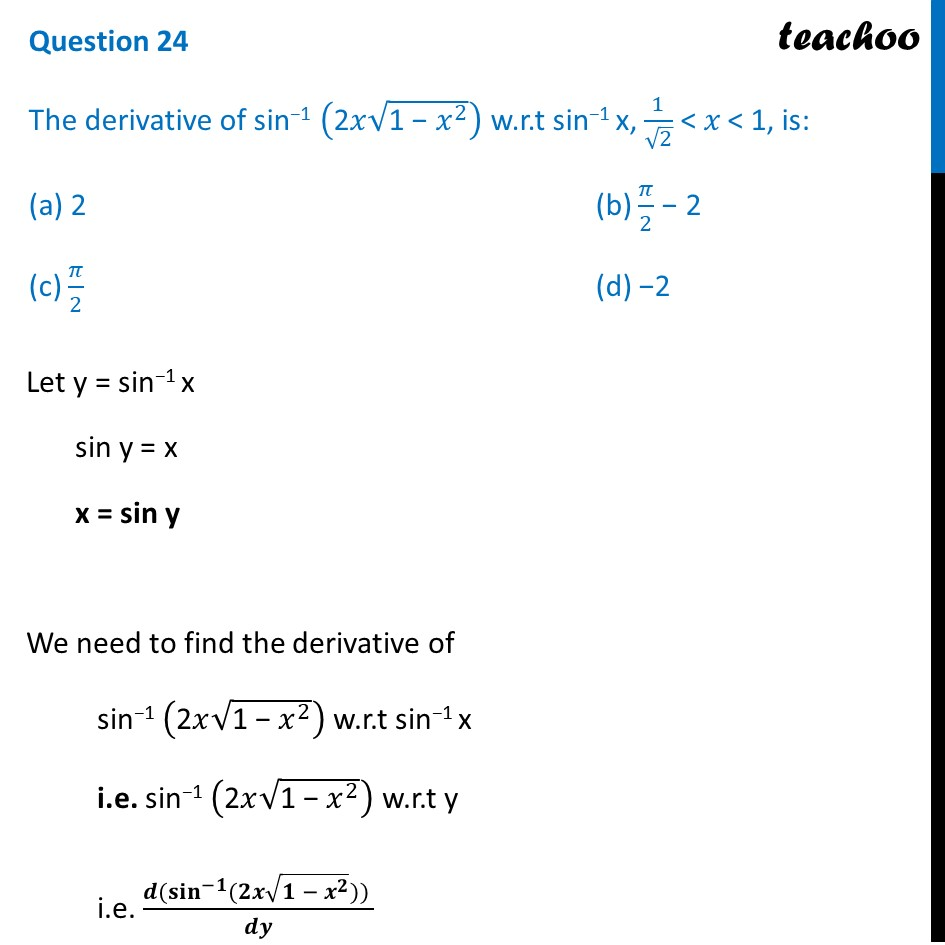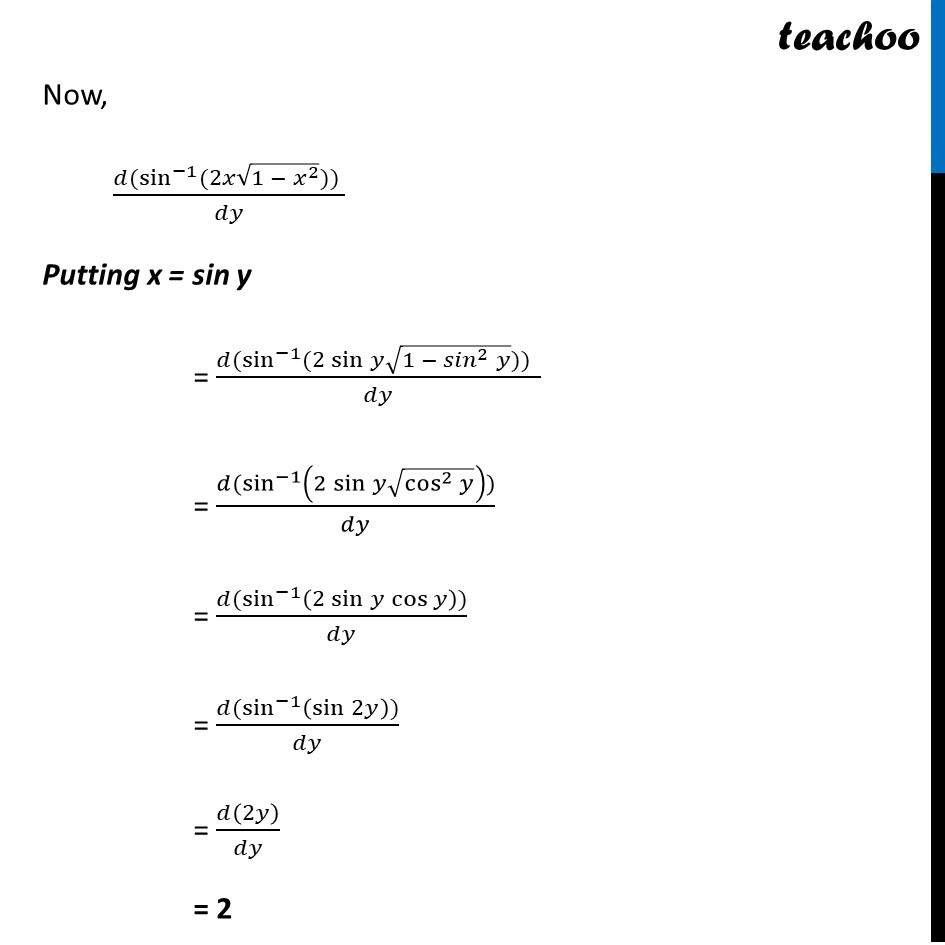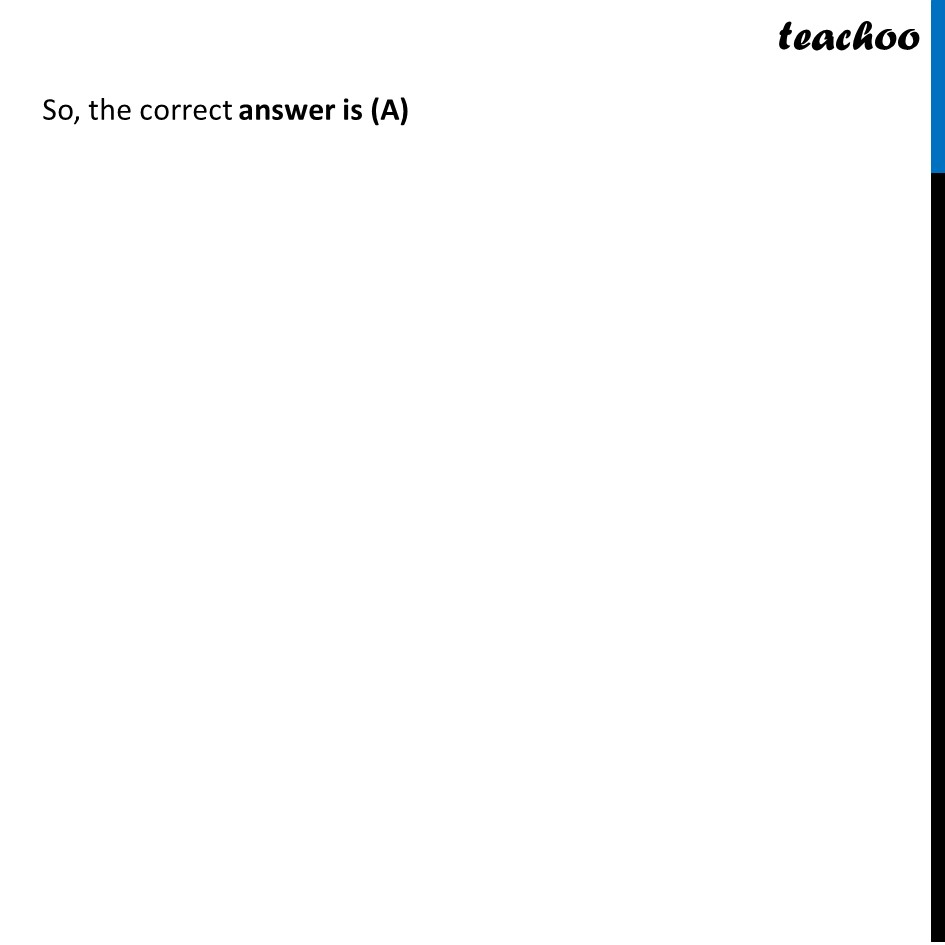CBSE Class 12 Sample Paper for 2022 Boards (MCQ Based - for Term 1)

Class 12
Solutions of Sample Papers and Past Year Papers - for Class 12 Boards

## (a) 2                 (b) π/2 − 2 (c) π/2              (d) −2Learn in your speed, with individual attention - Teachoo Maths 1-on-1 Class

### Transcript

Question 24 The derivative of sin−1 ("2𝑥" √("1 − " 𝑥^2 )) w.r.t sin−1 x, 1/√2 < 𝑥 < 1, is: (a) 2 (b) 𝜋/2 − 2 (c) 𝜋/2 (d) −2 Let y = sin−1 x sin y = x x = sin y We need to find the derivative of sin−1 ("2𝑥" √("1 − " 𝑥^2 )) w.r.t sin−1 x i.e. sin−1 ("2𝑥" √("1 − " 𝑥^2 )) w.r.t y i.e. (𝒅(〖𝐬𝐢𝐧〗^(−𝟏)⁡〖(𝟐𝒙√(𝟏 − 𝒙^𝟐 ))) 〗)/𝒅𝒚 Now, (𝑑(sin^(−1)⁡〖(2𝑥√(1 − 𝑥^2 ))) 〗)/𝑑𝑦 Putting x = sin y = (𝑑(sin^(−1)⁡〖(2 sin⁡𝑦 √(1 − 〖𝑠𝑖𝑛〗^2 𝑦))) 〗)/𝑑𝑦 = (𝑑(sin^(−1)⁡〖(2 sin⁡𝑦 √(cos^2⁡𝑦 )))〗)/𝑑𝑦 = (𝑑(sin^(−1)⁡〖(2 sin⁡𝑦 cos⁡𝑦 ))〗)/𝑑𝑦 = (𝑑(sin^(−1)⁡〖(sin⁡2𝑦 ))〗)/𝑑𝑦 = 𝑑(2𝑦)/𝑑𝑦 = 2 So, the correct answer is (A)